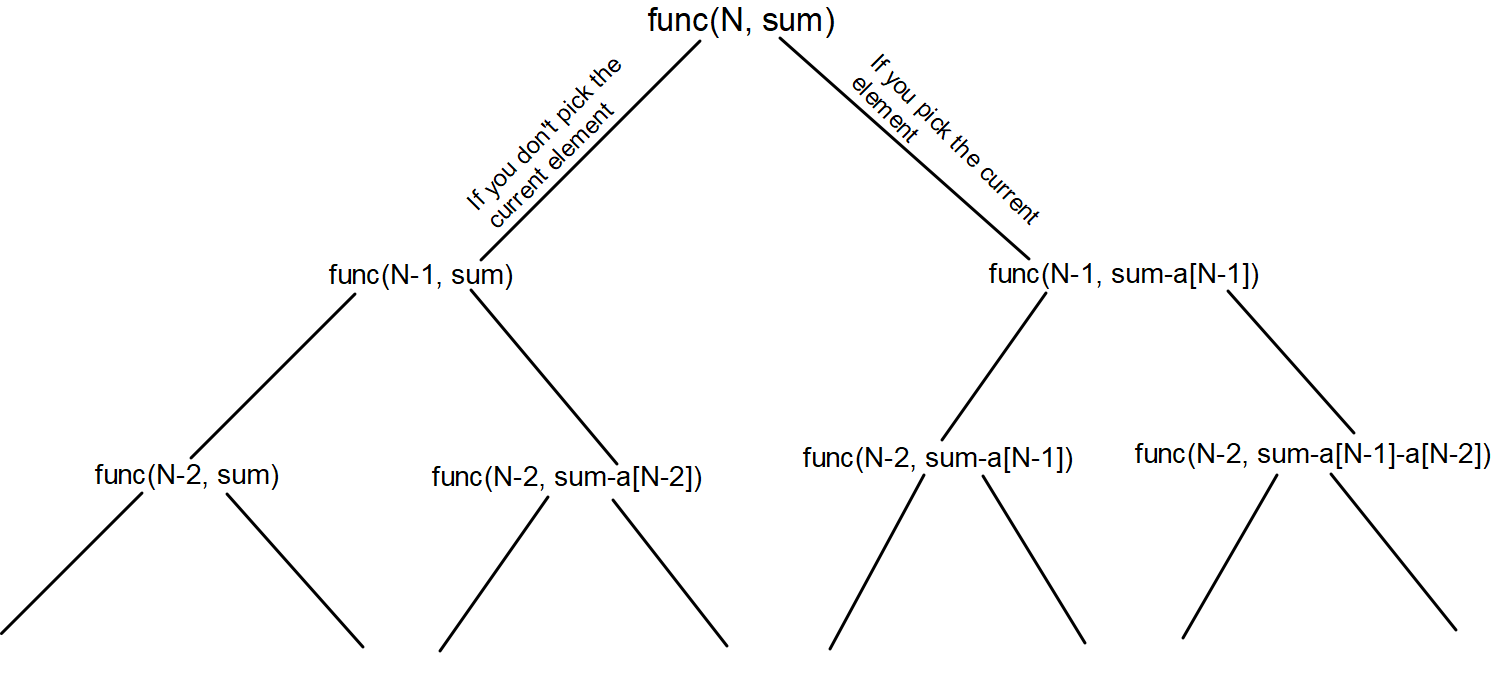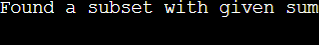# Subset Sum Problem

## Introduction:

You are given an array of non-negative integers and an integer value ‘target’. You need to search if there is a subset whose sum is equal to ‘target’.

Let us see few examples:

Input:

arr: {1, 2, 3, 4, 5}

target: 10

Output: True

Explanation:

{1, 4, 5} is one of the subsets of the input array whose subset sum is equal to the target value i.e., 10.

Input:

arr: {3, 7, 12, 2, 5}

target: 11

Output: False

Explanation:

There is no subset whose subset sum is equal to the given target value.

## APPROACH 1:

We can use Recursion here to solve this problem.

We can use the pick and non-pick strategy here to search for a subset whose sum is equal to the given target value. We can start from the ‘i’ = 0 index and make two moves, either picking the current element or leaving the current element.

If you pick the current element, add its value to the sum variable you are maintaining for each recursive call else, don’t add and call the recursive function for the ‘i + 1th’ index. When your ‘i’ reaches the value ‘N’ ( where ‘N’ is the total number of elements in the array), just check if your sum is equal to the target value or not.

Refer to the below recursive tree.Do refer to the below implementation of the above approach.

``````#include <iostream>
using namespace std;

/*
Returns true if there is a subset
of input arr with subset sum equal to given sum
*/
bool isSubsetSum(int arr[], int i, int sum, int target, int n)
{

// Base Cases
if(i == n){
return sum == target;
}
if (sum > target){
return false;
}
if (sum == target){
return true;
}

/*
Applying the pick and non-pick approach.
We will try both the cases to include or not
include the current element in the subset sum.
*/
return isSubsetSum(arr, i + 1, sum, target, n) || isSubsetSum(arr, i + 1, sum + arr[i], target, n);
}

// Driver code
int main()
{
int arr[] = {1, 2, 3, 4, 5};
int target = 50;
int n = sizeof(arr) / sizeof(arr);

if (isSubsetSum(arr, 0, 0, target, n) == true){
cout <<"Found a subset with given sum";
}
else{
cout <<"No subset with given sum";
}
return 0;
}``````

OUTPUT:Time Complexity: The time complexity for the approach is 2ⁿ because for each element we are making two decisions either to pick the current element or not to pick the current element.

Space Complexity: The space complexity for the above approach is constant. We are not using any auxiliary space.

## APPROACH 2:

We can optimize APPROACH 1 by memoizing it. We can create a 2D D.P. table of size (arr.size() + 1) * (target + 1) to store the answer for each ‘i’ and ‘sum’ values because it might be possible that we try to compute the answer for the same ‘i’ and ‘sum’ values.

Refer to the below implementation for the above optimization.

``````#include <bits/stdc++.h>
using namespace std;

//Making the dp table global
int dp;

/*
Returns true if there is a subset
of input arr with subset sum equal to given sum
*/
int isSubsetSum(int arr[], int i, int sum, int target, int n)
{

// Base Cases
if(i == n){
return sum == target;
}
if (sum > target){
return 0;
}
if (sum == target){
return 1;
}

/*
If the answer for current state
*/
if(dp[i][sum] != -1){
return dp[i][sum];
}

/*
Applying the pick and non-pick approach.
We will try both the cases to include or not
include the current element in our subset sum.
*/
return dp[i][sum] = isSubsetSum(arr, i + 1, sum, target, n) || isSubsetSum(arr, i + 1, sum + arr[i], target, n);
}

// Driver code
int main()
{
int arr[] = {1, 2, 3, 4, 5};
int target = 10;
int n = sizeof(arr) / sizeof(arr);

// Storing the value -1 to the dp table
memset(dp, -1, sizeof(dp));

if (isSubsetSum(arr, 0, 0, target, n)){
cout <<"Found a subset with given sum";
}
else{
cout <<"No subset with given sum";
}
return 0;
}``````

OUTPUT:Time Complexity: The time complexity for the above approach is (arr.size() + 1) * (target + 1) because we are storing the answer for all possible ‘i’ and ‘sum’ pairs, and if we have already calculated the answer for any recursion call we return its answer stores in the DP table.

Space Complexity: The space complexity for the above approach is (arr.size() + 1) * (target + 1) because we are creating a DP table of size (arr.size() + 1) * (target + 1) to store the answer for different ‘i’ and ‘sum’ pairs.

## APPROACH 3:

We can solve the above recursive approach in an iterative manner using bottom-up D.P.

We can create a 2D D.P. similar to the second approach of size (target + 1) * (arr.size() + 1) to store the answer for each ‘i’ and ‘sum’ values.

``````#include <iostream>
using namespace std;

/*
Returns true if there is a subset of
a[] with sum equal to given sum
*/
bool isSubsetSum(int a[], int n, int sum)
{

/*
The value of dp[i][j] will be
true if there is a subset of
a[0..j-1] with sum equal to i
*/
bool dp[n + 1][sum + 1];

// If sum is 0, then answer is true
for (int i = 0; i <= n; i++)
dp[i] = true;

/*
If sum is not 0 and set is empty,
*/
for (int i = 1; i <= sum; i++)
dp[i] = false;

/*
Fill the dp table in bottom
up manner
*/
for (int i = 1; i <= sum; i++) {
for (int j = 1; j <= n; j++) {
dp[i][j] = dp[i][j - 1];
if (i >= a[j - 1]){
dp[i][j] = dp[i][j] || dp[i - a[j - 1]][j - 1];
}
}
}

return dp[sum][n];
}

int main()
{
int a[] = { 1, 2, 3, 4, 5 };
int sum = 7;
int n = sizeof(a) / sizeof(a);

if (isSubsetSum(a, n, sum) == true){
cout <<"Found a subset with given sum";
}
else{
cout <<"No subset with given sum";
}
return 0;
}``````

OUTPUT:Time Complexity: The time complexity for the above approach is (arr.size() + 1) * (target + 1) because we are storing the answer for all possible ‘i’ and ‘sum’ pairs.

Space Complexity: The space complexity for the above approach is (arr.size() + 1) * (target + 1) because we are creating a DP table of size (arr.size() + 1) * (target + 1) to store the answer for different ‘i’ and ‘sum’ pairs.

To better understand and implement the code, check out this video for both the recursive and dynamic programming solutions.

## FAQs:

1. What optimization did we do on the recursive approach for the Subset Sum problem?
• Since we were computing the answer for the same state at different times, we made a DP table to store the answer for the states we already computed, so that if in the future we need the answer for that state we can directly return the answer from the DP table.
2. What is the time complexity for the optimized approach?
• The time complexity for the optimized approach is (arr.size() + 1) * (target + 1) because we are storing the answer for all possible ‘i’ and ‘sum’ pairs, and if we have already calculated the answer for any recursion call we return its answer stores in the DP table.
3. Can we apply a similar approach if both the array length and the target value have their maximum value of 10⁵?
• No, if we apply a similar approach for the mentioned constraints, the compiler will give us the ‘Memory Limit Exceeded’ error because of forming the DP table.

## Key Takeaways:

In this blog we have covered the following things:

1. We first discussed the Recursive approach to solve this problem.
2. Then we saw how we optimized the approach by memoizing using the DP table.

If you want to learn more about Arrays and want to practice some questions which require you to take your basic knowledge on Arrays a notch higher, then you can visit our Guided Path for Arrays. To practice this problem you can visit Practice Problem.

Until then, All the best for your future endeavors, and Keep Coding.